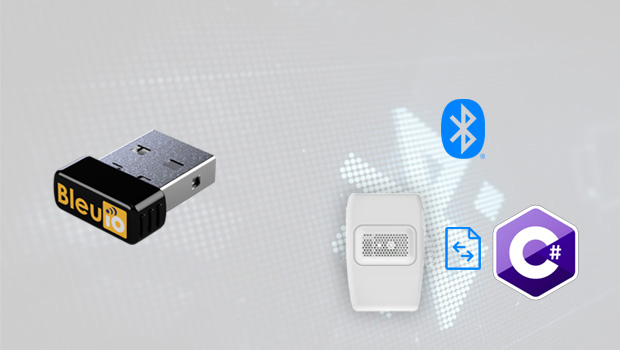# C# Desktop application to get real-time BLE data from Air Quality Sensor

January 19, 2022Bluetooth Low Energy (BLE) is a low power wireless technology used to connect devices. It is a popular communication method, especially in the Internet of Things era. Several devices around the house have a built-in Bluetooth transceiver, and most of them provide useful capabilities to automate jobs. This technology is widely used in the healthcare, fitness, beacons, security, and home entertainment industries. For that reason, it is really interesting to create a desktop application using C# that access BLE device data around the house.

In this example, we will create a simple C# windows form application to get real-time HibouAir environmental data using BleuIO.

This script can be used to connect and view other BLE devices data.

Let’s start

First, let’s create a new project in visual studio and select C# windows form application from the list.

Choose a suitable name for your project.

Once the project is created, we will see a blank form screen where we will add buttons and labels to communicate with BleuIO graphically.

The application will connect to the BleuIO dongle to its given COM port from the script. You can change the port easily by going to line number 18.
We will have a disconnect button to disconnect BleuIO from the COM port.

The button Scan for HibouAir devices will look for nearby HibouAir devices and store them in an Array. The Combobox (dropdown item) next to it will load the stored device from where we can select a device to get its data.

The Get data button will show the real-time environmental data of this device.

The form will look like this.

The .cs file associated to this will have the following code.

Source code is available at https://github.com/smart-sensor-devices-ab/bluetooth_realtimedata_csharp

``````using System;
using System.IO.Ports;
using System.Linq;
using System.Text;
using System.Windows.Forms;
using System.Collections;

namespace WindowsFormsApp1
{

public partial class Form1 : Form
{
//Connect to Serial port
//Replace port number to your comport
SerialPort mySerialPort = new SerialPort("COM18", 57600, Parity.None, 8, StopBits.One);
ArrayList device = new ArrayList();
string ScannedData = "";
public string selectedDevices = "";

// HibouAir Advertise data decrypt and show output
public String ParseSensorData(string input)
{
int counter = 17;
string theData = " SensorID : " + input[counter + 1] +
input[counter + 2] +
input[counter + 3] +
input[counter + 4] +
input[counter + 5] +
input[counter + 6] + "\n\n " +
"Pressure : " + Convert.ToInt32(input[counter + 13].ToString() + input[counter + 14].ToString() + input[counter + 11].ToString() + input[counter + 12].ToString(), 16) / 10 + " mbar\n\n " +
"Temperature : " + Convert.ToInt32(input[counter + 17].ToString() + input[counter + 18].ToString() + input[counter + 15].ToString() + input[counter + 16].ToString(), 16) / 10 + " °C\n\n " +
"Humidity : " + Convert.ToInt32(input[counter + 21].ToString() + input[counter + 22].ToString() + input[counter + 19].ToString() + input[counter + 20].ToString(), 16) / 10 + " %rH\n\n " +
"VOC : " + Convert.ToInt32(input[counter + 25].ToString() + input[counter + 26].ToString() + input[counter + 23].ToString() + input[counter + 24].ToString(), 16) / 10 + "\n\n " +
"ALS : " + Convert.ToInt32(input[counter + 9].ToString() + input[counter + 10].ToString() + input[counter + 7].ToString() + input[counter + 8].ToString(), 16) + " Lux\n\n " +
"PM 1.0 : " + Convert.ToInt32(input[counter + 29].ToString() + input[counter + 30].ToString() + input[counter + 27].ToString() + input[counter + 28].ToString(), 16) / 10 + " µg/m³\n\n " +
"PM 2.5 : " + Convert.ToInt32(input[counter + 33].ToString() + input[counter + 34].ToString() + input[counter + 31].ToString() + input[counter + 32].ToString(), 16) / 10 + " µg/m³\n\n " +
"PM 10 : " + Convert.ToInt32(input[counter + 37].ToString() + input[counter + 38].ToString() + input[counter + 35].ToString() + input[counter + 36].ToString(), 16) / 10 + " µg/m³"
;

sensor_op.Text = theData.ToString();
return theData;
}

public Form1()
{
InitializeComponent();
mySerialPort.Open();
ArrayList device = new ArrayList();

}

//Store response from the dongle
{

SerialPort sp = (SerialPort)sender;
{

}
if (selectedDevices != "")
{
label1.Invoke(new EventHandler(delegate { label1.Text = selectedDevices.Remove(0, 3); }));

{
ScannedData=s;

// output_data.Invoke(new EventHandler(delegate { output_data.Text += "TT: " + s + "\r\n"; }));
}
}
//output_data.Invoke(new EventHandler(delegate { output_data.Text += s + "\r\n"; }));

//lbl_output.Invoke(this.myDelegate, new Object[] { s });
}

// Disconnect from COM port
private void btn_disconnect_Click(object sender, EventArgs e)
{
mySerialPort.Close();
Environment.Exit(0);
}

private void Form1_Load(object sender, EventArgs e)
{

}

private void pictureBox1_Click(object sender, EventArgs e)
{

}

private void btn_deviceList_Click(object sender, EventArgs e)
{
var inputByte = new byte[] { 13 };
byte[] dualCmd = Encoding.UTF8.GetBytes("AT+DUAL");
dualCmd = dualCmd.Concat(inputByte).ToArray();
mySerialPort.Write(dualCmd, 0, dualCmd.Length);

byte[] gapScanCmd = Encoding.UTF8.GetBytes("AT+GAPSCAN=2");
gapScanCmd = gapScanCmd.Concat(inputByte).ToArray();
mySerialPort.Write(gapScanCmd, 0, gapScanCmd.Length);

}

private void label1_Click(object sender, EventArgs e)
{

}

private void dropdown_device_SelectedIndexChanged(object sender, EventArgs e)
{
{

foreach (var a in device)
{
}
}
else
{
selectedDevices = dropdown_device.Text;
string[] selectedDeviceID = selectedDevices.Split(' ');
selectedDevices = selectedDeviceID;
btnGetData.Visible = true;
}

}

//get advertised data of selected device
private void btnGetData_Click(object sender, EventArgs e)
{

var inputByte = new byte[] { 13 };
byte[] gapScanCmd = Encoding.UTF8.GetBytes("AT+FINDSCANDATA=FF5B07");
gapScanCmd = gapScanCmd.Concat(inputByte).ToArray();
mySerialPort.Write(gapScanCmd, 0, gapScanCmd.Length);
byte[] bytes = Encoding.UTF8.GetBytes("\u0003");
bytes = bytes.Concat(inputByte).ToArray();
mySerialPort.Write(bytes, 0, bytes.Length);
var array = ScannedData.Split('\n');
int i = 0;
//filter selected device data
foreach (var a in array)
{
string kk = (string)a;
if (kk.Contains(selectedDevices.Remove(0, 3)))
{
var bleData = array[i].Split(' ');

break;
}

i++;

}

}
}

}
``````

As you can notice, I wrote COM18 to connect to the serial port because the BleuIO device on my computer is connected to COM18.
You can check your COM port from the device manager.
Let’s run the project and click on scan for HibouAir devices.

Here is a demo of this sample application.